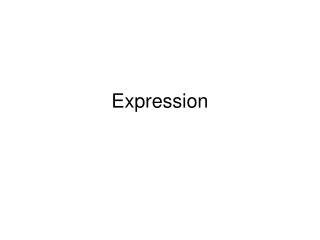DownloadDownload PresentationExpression

# Expression

Download Presentation## Expression

- - - - - - - - - - - - - - - - - - - - - - - - - - - E N D - - - - - - - - - - - - - - - - - - - - - - - - - - -
##### Presentation Transcript

1. Expression

2. Arithmetic Operators • +, -, *, /, ^, ( ) • Evaluating an expression • Examples: • 4 + 2 – 1 • 4 + 2 * 3 • 3 – 4 / 2 • 8 / 4 * 2 • 2 * 3 ^ 2 • (2 * 3) + 2 ^ 3

3. Order of Evaluation • Operator Multiples • 1 ( ) Inner to outer, left to right • 2. ^ left to right • 3. *, / left to right • 4. +, - left to right

4. Formula to Expression

5. ((A+B))*2

6. Expression to Formula • A / B * C - 2 • A + B * C ^ D • (A + B / (C – 2)) ^ D + 1 • Evaluating an expression: • 4 * 2 + 8 / 4 * 2 ^ 2 – 1 • 18 / 3 ^ 2 * (1 + 2)

7. Example Note: PV = f (FutureValue, IntRate, Years) Independent variables, dependent variable

8. Text Operator • Concatenation: & • Join two or more text values into a single text value.

9. Number and Text Are Not Compatible • ‘1 is different from 1. • #VALUE • Blank and space are different.

10. Data Representation • Digitizing: • Data, image, video, music • Digital smell • Binary digit: Bit • 0, 1

11. Text Data • Text (Character, String) • Letters, digits, other symbols • Coding schemes: • ASCII: 8 bits (byte), 256 characters • “1” : 0110 0001 • The code for “A” is different from “a”. • EBCDIC: 8 bits • UniCode: 16 bits, 65536 characters

12. Numeric Data • Integer: Binary number • 1: 0000 0001 • Real: Floating number • 123.456 -> .123456 * 10^3

13. Case Sensitive/Insensitive • Many computer systems are case sensitive: • Operating systems: • Unix, Linux • Programming languages: • Java, C++, C# • Database: • XML • Demo: Telnet:libra.sfsu.edu • Username, Password

14. Comparing Text Data • Collating sequence: the ordering of characters: • Blank • Digits in numerical order • Upper case letters in alphabetical order • Lower case letters in alphabetical order • To compare two texts: • From left to right • Character for character

15. Examples • “JEAN” < “JOHN”: True/False • “John” < “Johny”: True/False • “1234” < “45”: True/False • Arrange “alan”, “Chao”, “JOHN” in ascending order: • Arrange P1, P3, P10, P15, P20 in ascending order: • Oracle database demo: Telnet: libra.sfsu.edu • Select * from Cust; • Select * from Cust Order by Cname;

16. Excel is Case Insensitive • Order: • Blank • Digits in numerical order • Letters in alphabetical order • Data/Sort/Options/Case Sensitive: • a<A<b<B<c<C …..